Examples

Chapter 5 Class 10 Arithmetic Progressions
Serial order wise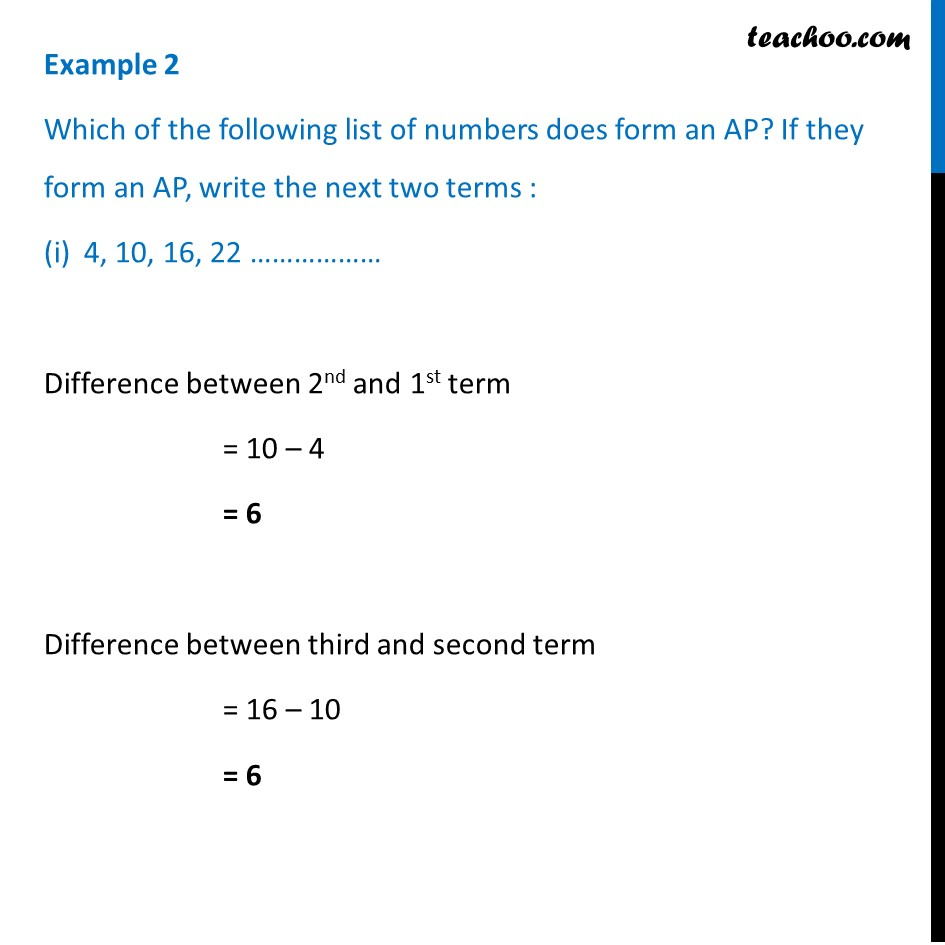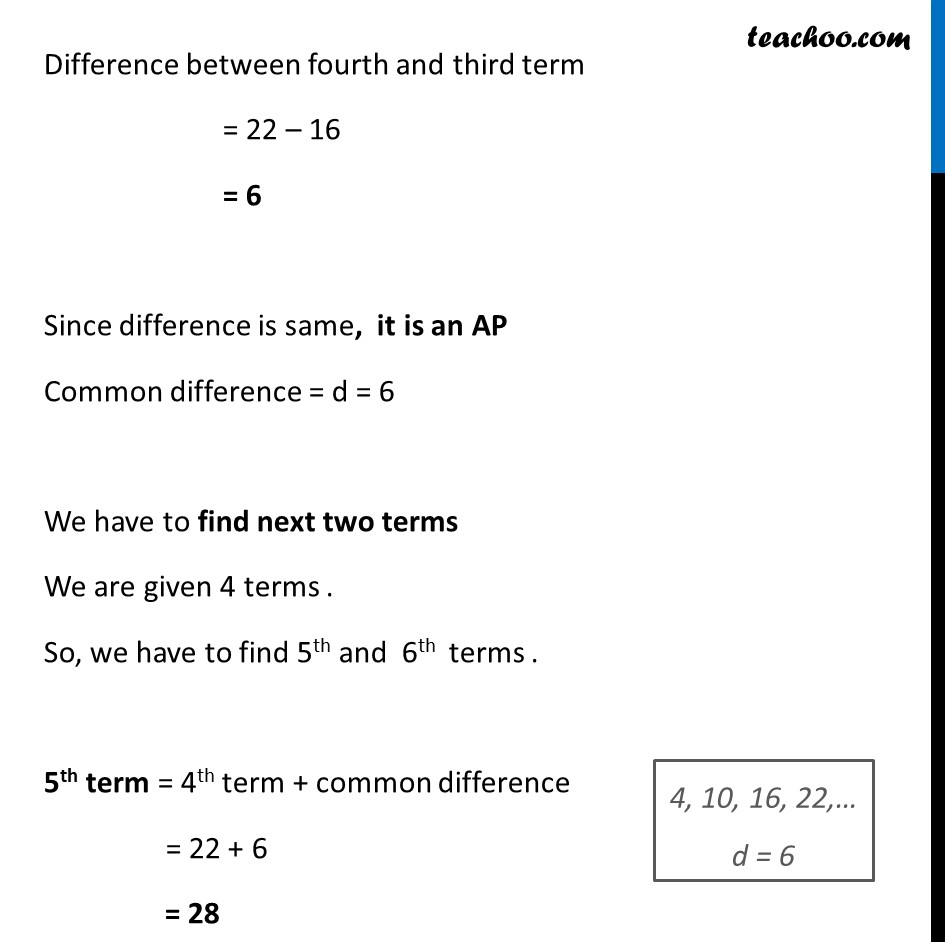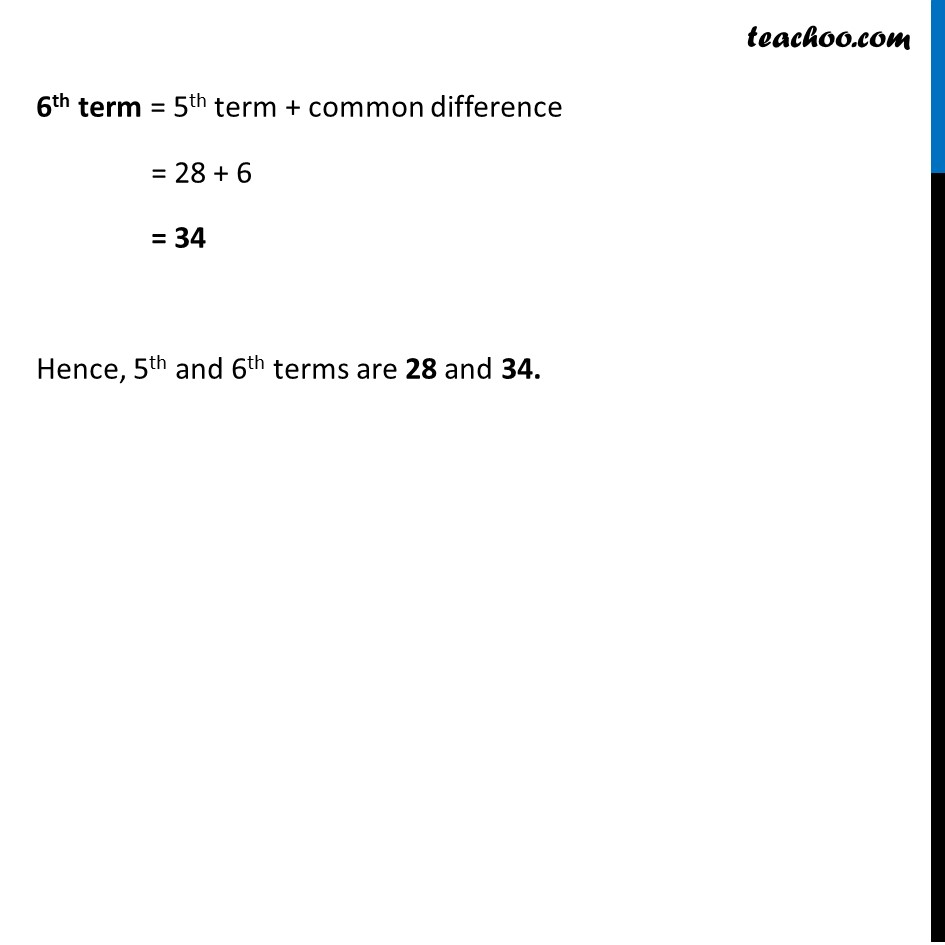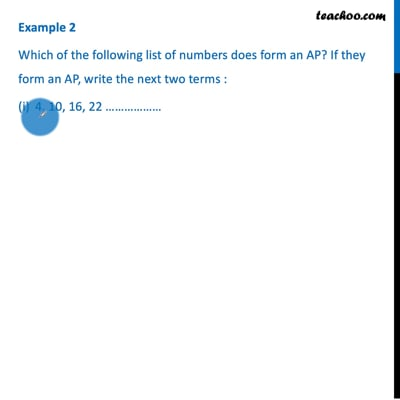This video is only available for Teachoo black users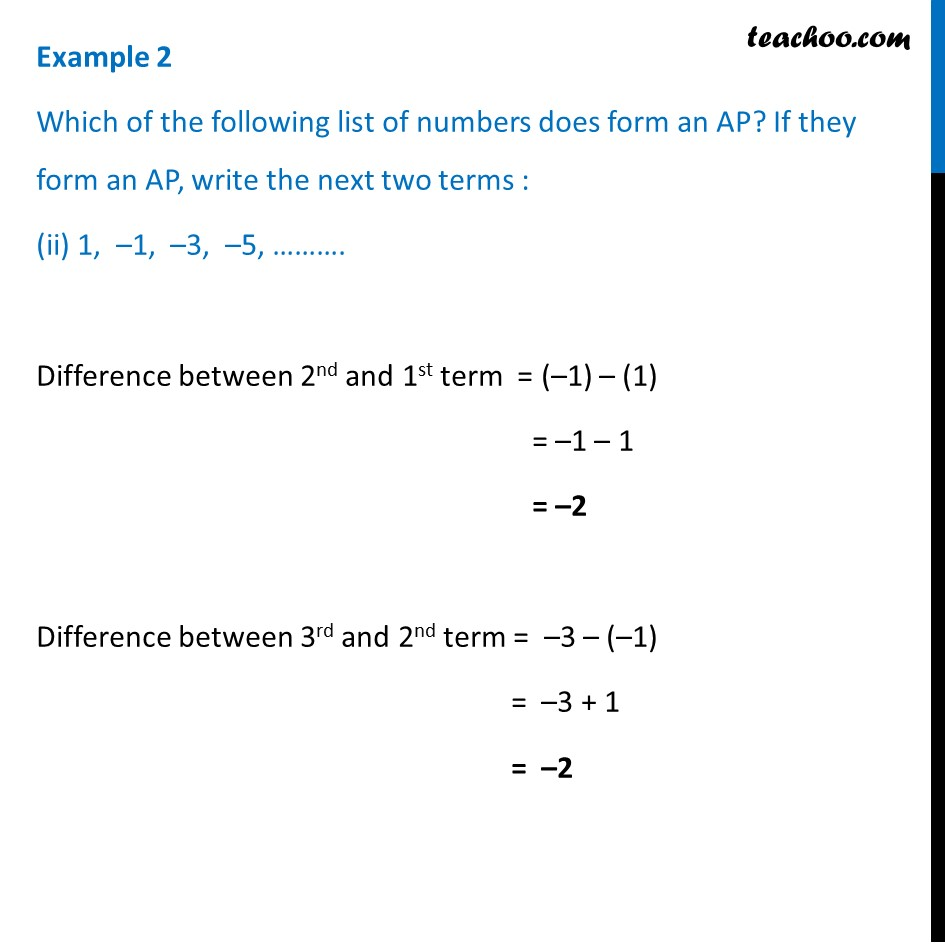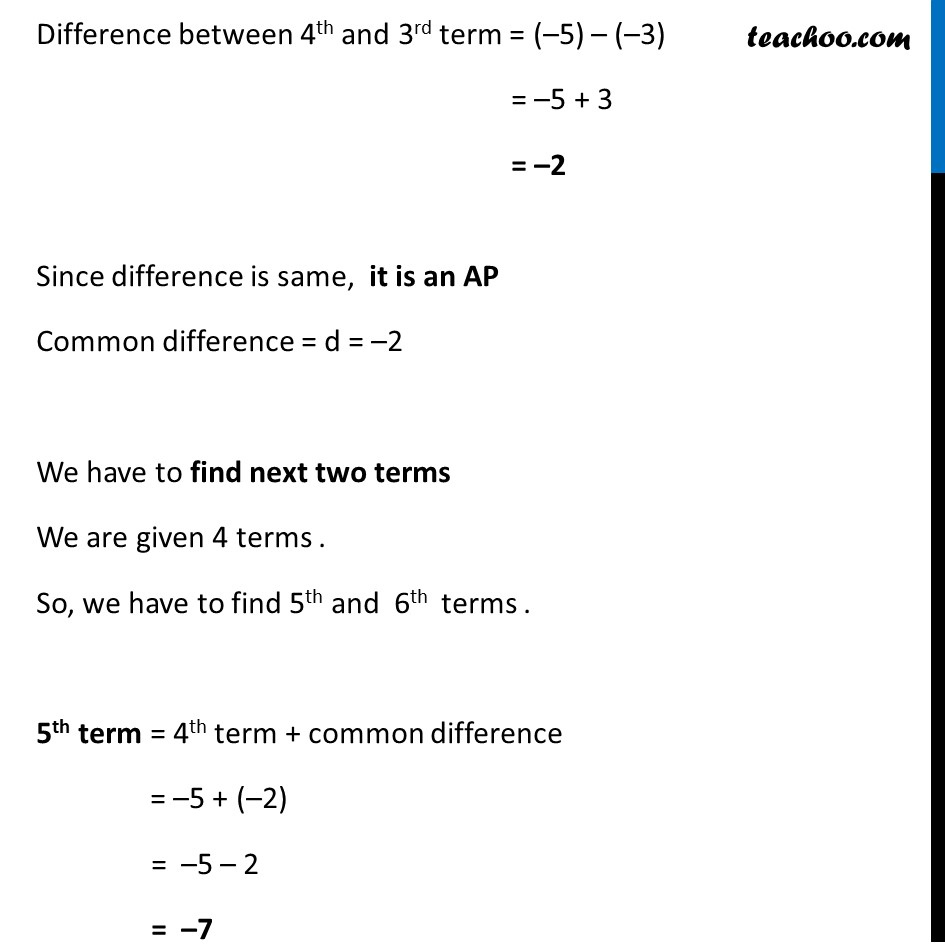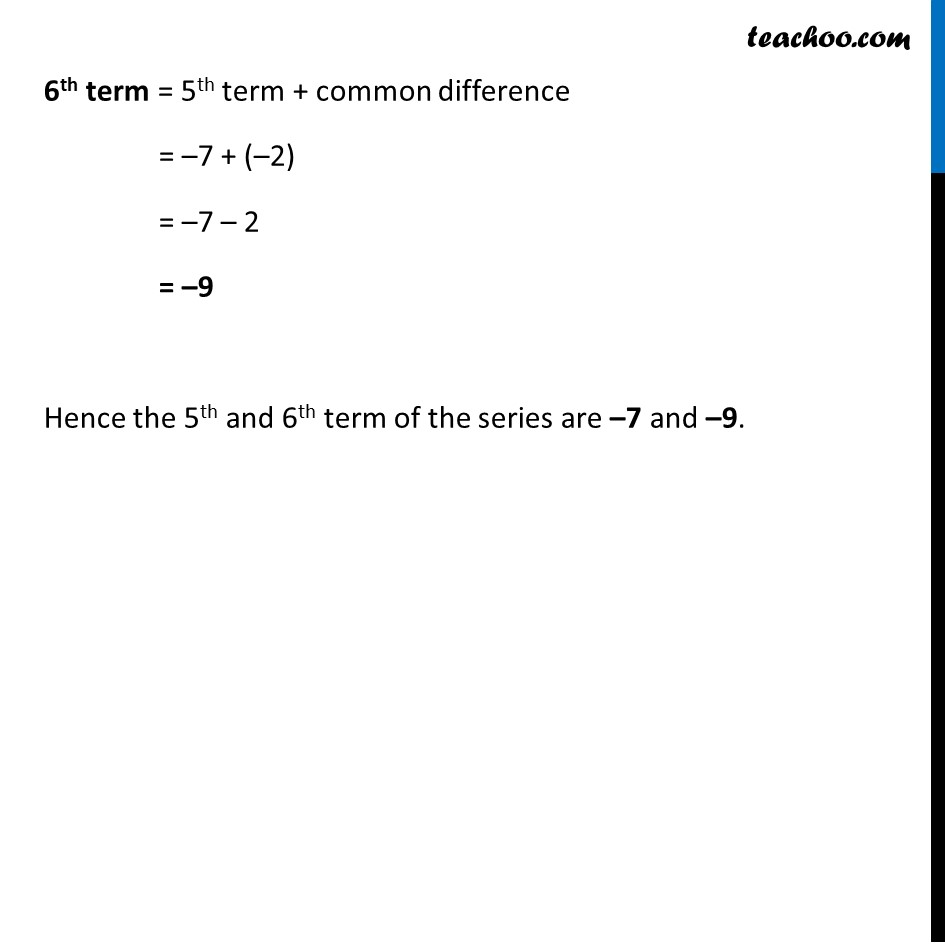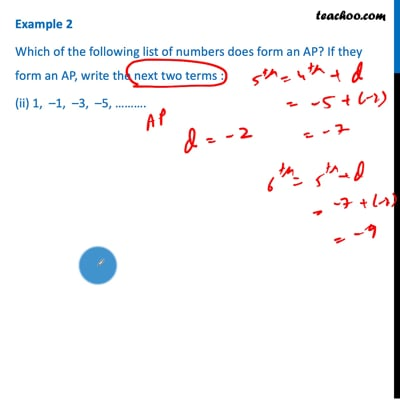This video is only available for Teachoo black users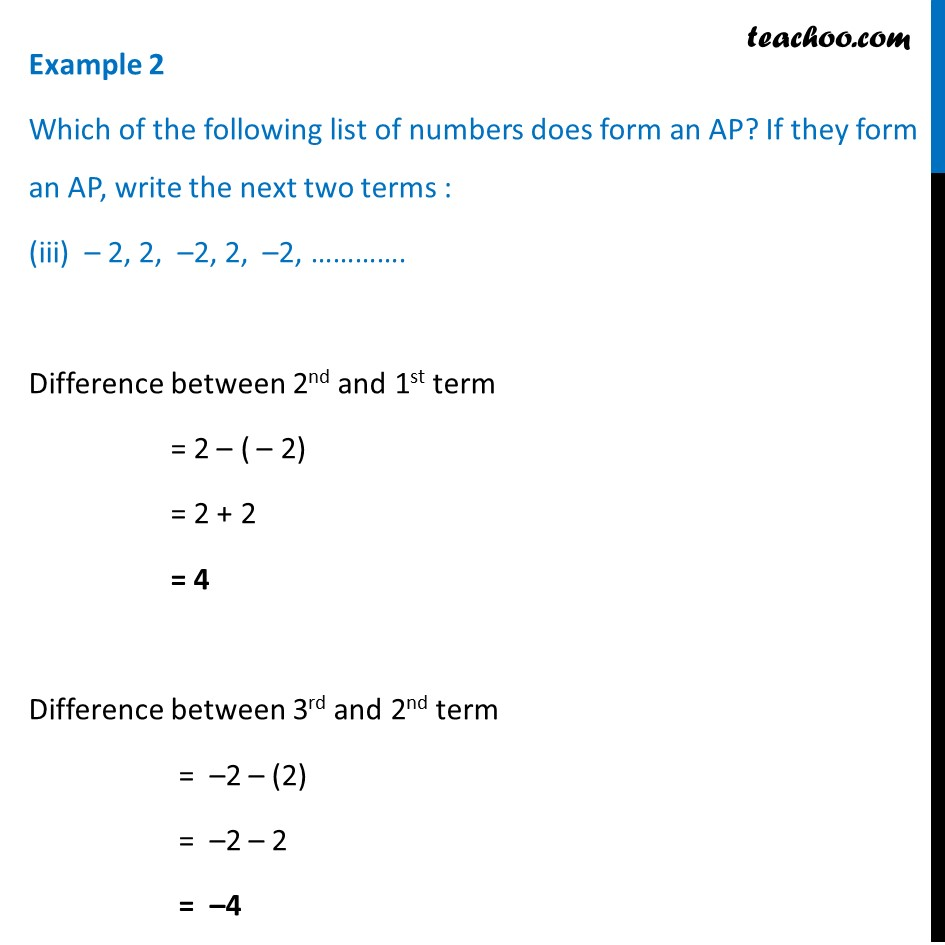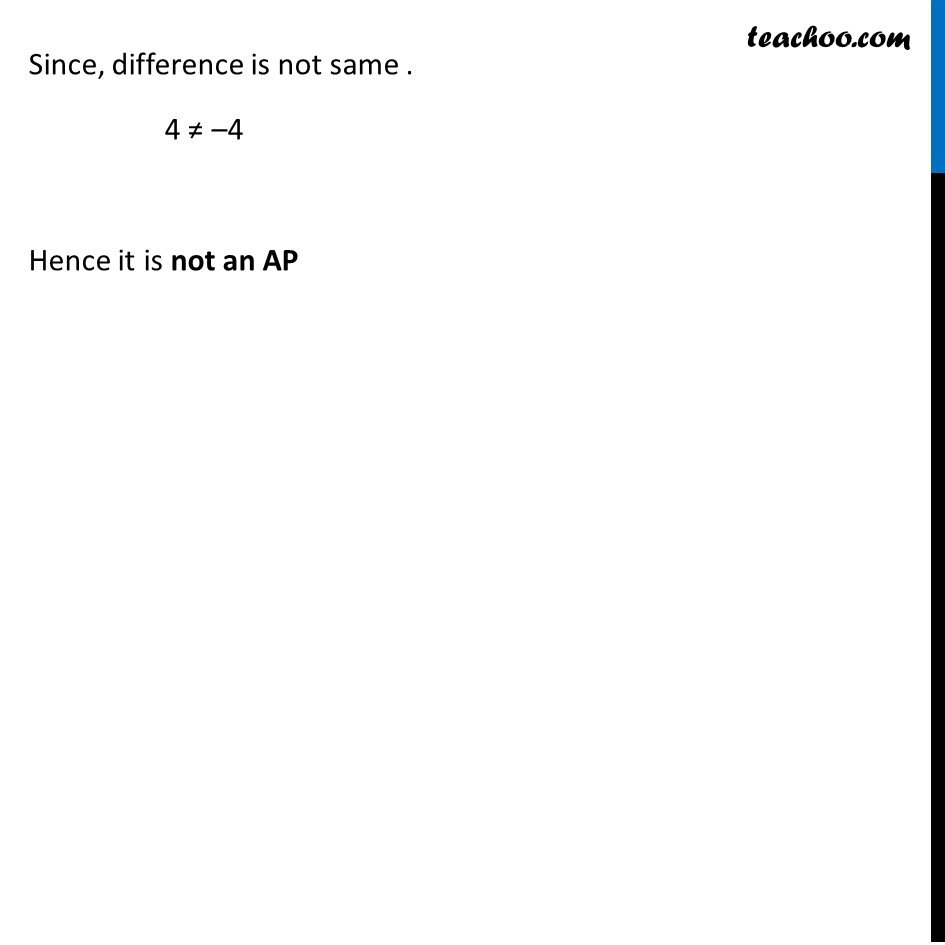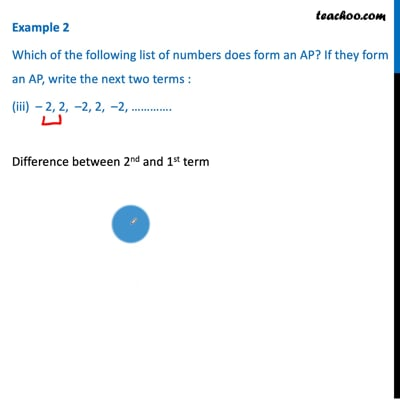This video is only available for Teachoo black users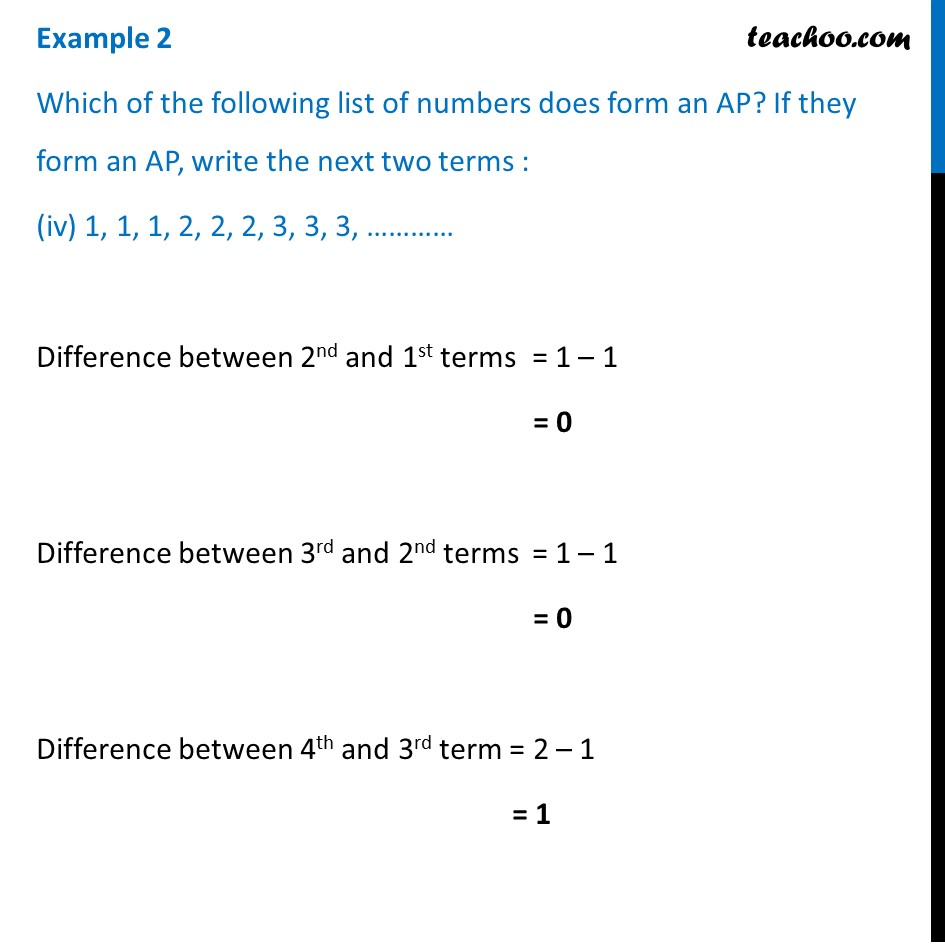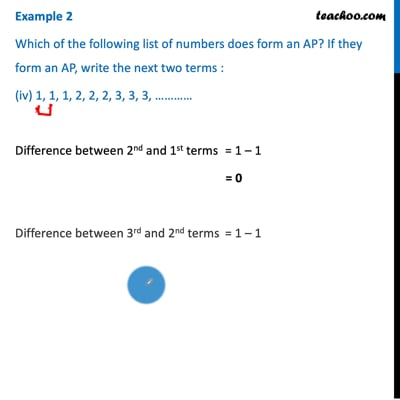This video is only available for Teachoo black users

Solve all your doubts with Teachoo Black (new monthly pack available now!)

### Transcript

Example 2 Which of the following list of numbers does form an AP? If they form an AP, write the next two terms : 4, 10, 16, 22 ……………… Difference between 2nd and 1st term = 10 – 4 = 6 Difference between third and second term = 16 – 10 = 6 Difference between fourth and third term = 22 – 16 = 6 Since difference is same, it is an AP Common difference = d = 6 We have to find next two terms We are given 4 terms . So, we have to find 5th and 6th terms . 5th term = 4th term + common difference = 22 + 6 = 28 4, 10, 16, 22,… d = 6Difference between fourth and third term = 22 – 16 = 6 Since difference is same, it is an AP Common difference = d = 6 We have to find next two terms We are given 4 terms . So, we have to find 5th and 6th terms . 5th term = 4th term + common difference = 22 + 6 = 28 4, 10, 16, 22,… d = 6 6th term = 5th term + common difference = 28 + 6 = 34 Hence, 5th and 6th terms are 28 and 34. Example 2 Which of the following list of numbers does form an AP? If they form an AP, write the next two terms : (ii) 1, –1, –3, –5, ………. Difference between 2nd and 1st term = (–1) – (1) = –1 – 1 = –2 Difference between 3rd and 2nd term = –3 – (–1) = –3 + 1 = –2 Difference between 4th and 3rd term = (–5) – (–3) = –5 + 3 = –2 Since difference is same, it is an AP Common difference = d = –2 We have to find next two terms We are given 4 terms . So, we have to find 5th and 6th terms . 5th term = 4th term + common difference = –5 + (–2) = –5 – 2 = –7 6th term = 5th term + common difference = –7 + (–2) = –7 – 2 = –9 Hence the 5th and 6th term of the series are –7 and –9. Example 2 Which of the following list of numbers does form an AP? If they form an AP, write the next two terms : (iii) – 2, 2, –2, 2, –2, …………. Difference between 2nd and 1st term = 2 – ( – 2) = 2 + 2 = 4 Difference between 3rd and 2nd term = –2 – (2) = –2 – 2 = –4 Since, difference is not same . 4 ≠ –4 Hence it is not an AP Example 2 Which of the following list of numbers does form an AP? If they form an AP, write the next two terms : (iv) 1, 1, 1, 2, 2, 2, 3, 3, 3, ………… Difference between 2nd and 1st terms = 1 – 1 = 0 Difference between 3rd and 2nd terms = 1 – 1 = 0 Difference between 4th and 3rd term = 2 – 1 = 1 Since difference is not same 0 ≠1 Hence it is not an AP. Since difference is not same 0 ≠1 Hence it is not an AP.